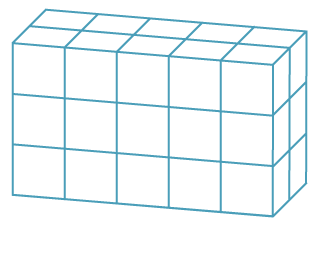### Associative law for multiplication

Multiplying three whole numbers corresponds geometrically to calculating the number of unit cubes in (or volume of) a rectangular prism.The volume is given by (5 × 2) × 3 = 5 × (2 × 3).

This is the associative law for multiplication.

The left-hand side of the equality is (5 × 2) × 3 = 10 × 3 = 30.

The right-hand side of the equality is 5 × (2 × 3) = 5 × 6 = 30.

It does not matter if you multiply the first two first or the second two numbers first.